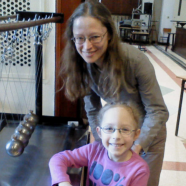# Joanna Raczek - Science profile - MOST Wiedzy

## Search## dr inż. Joanna Raczek

### Contact

Workplace
Budynek A Elektroniki
pokój EA 247 open in new tab
Phone
+ 48 58 347 15 64
E-mail
joanna.raczek@pg.edu.pl

### Publication showcase

• #### Polynomial Algorithm for Minimal (1,2)-Dominating Set in Networks

- Year 2022

Dominating sets find application in a variety of networks. A subset of nodes D is a (1,2)-dominating set in a graph G=(V,E) if every node not in D is adjacent to a node in D and is also at most a distance of 2 to another node from D. In networks, (1,2)-dominating sets have a higher fault tolerance and provide a higher reliability of services in case of failure. However, finding such the smallest set is NP-hard. In this paper, we...

• #### Progress on Roman and Weakly Connected Roman Graphs

- Year 2021

A graph G for which γR(G)=2γ(G) is the Roman graph, and if γwcR(G)=2γwc(G), then G is the weakly connected Roman graph. In this paper, we show that the decision problem of whether a bipartite graph is Roman is a co-NP-hard problem. Next, we prove similar results for weakly connected Roman graphs. We also study Roman trees improving the result of M.A. Henning’s A characterization of Roman trees, Discuss. Math. Graph Theory 22 (2002)....

• #### Block graphs with large paired domination multisubdivision number

- Year 2021

The paired domination multisubdivision number of a nonempty graph G, denoted by msdpr(G), is the smallest positive integer k such that there exists an edge which must be subdivided k times to increase the paired domination number of G. It is known that msdpr(G) ≤ 4 for all graphs G. We characterize block graphs with msdpr(G) = 4.